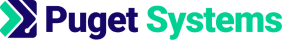https://www.pugetsystems.com
Share:

Yes, Intel is doing their own Python build! It is still in beta but I think it's a great idea. Python is a pretty important programming language. It has a large and growing number of useful libraries for mathematical/scientific computing and machine learning, NumPy, SciPy, pandas, Scikit-learn, PySpark, theano, ...etc... Python is often used as a frontend or "glue code" language so most important API's get a nice Python interface at some point. You can find Python modules for just about everything. Yeah, it's important!

Python is not my favorite scripting language for mathematical programming. It is "C" like which makes it awkward for mathematical computing in my opinion. Yes, I like Fortran! To me a vector acts like a "column", n x 1 matrix, and its "first" element is v not v! Math is hard enough without having to fight your programming language to get indexing and logic correct in your algorithms. Languages that get things "right" are Julia, Lua, Mathematica, Matlab and Fortran. At least that's my opinion/rant! I do actually like Python but I just have to give that rant when I get a chance :-)

People are using Python for serious computing work so Intel's effort to do an optimized Python is significant. You can build Python with Intel compilers yourself and link stuff like NumPy to the Intel MKL. However, Intel has some really great compiler guys and I expect that they will get a highly optimized Python put together.

Asside: It makes good sense for Intel to work on a Python build but really it would be great if they were looking at Julia! Julia is the most promising and, possibly, the most important new language to come out in over a decade! http://julialang.org/

## Intel Python

You can register to get early access for Intel Python at, https://software.intel.com/en-us/python-distribution

You will need to register for the preview release and you will need to login to your Intel dev account, i.e. if you don't have an account at Intel you will need to create one. There are Python 2.7 and 3.5 builds for Linux and Windows. The email sent after registration contains a serial number and as says "The software license expires on April 29, 2016". The note in the email says you will need the serial number but the installer never asked for it. Be aware that this is "preview" stuff! The version I downloaded is tagged 1.0.0tp1 but the release notes are tagged v0.1. Be sure to read the release notes!

The version I downloaded untared to pythoni-2.7.20150803_184913. I ran the install script and installed in my home directory instead of doing a root install. There is an environment setup script bin/pythonvars.sh that you can source to set paths and such.

## Lets try it

I did a simple matrix multiply and computed the norm of the result using loops and with numpy functions. The code at the end of this post was run using the CentOS 7.2 default python and the Intel python preview.

## Here's the results:

Note: Using loops in python is ridiculously slow so I used a small matrix size for that and a much larger size for numpy. Keep in mind that matrix multiplication complexity increases as n cubed!

Note: I expected Intel's numpy to be fast but it is significant that plain old python code is much faster with the Intel version too.

### CentOS 7.2 default Python

```[kinghorn@i7 python-test]\$ python simple-py-test.py

2.7.5 (default, Nov 20 2015, 02:00:19)
[GCC 4.8.5 20150623 (Red Hat 4.8.5-4)]

matrix multiply n = 400    with loops  68.427585125 seconds
matrix norm     n = 400    with loops  0.135160923004 seconds
norm of C is                           40007.6355286

matrix multiply n = 10000  with numpy  36.3187189102 seconds
matrix norm     n = 10000  with numpy  0.324057102203 seconds
norm of C is                           25001868.2292

```

### Intel Python preview

```[kinghorn@i7 python-test]\$ source ~/opt/intel/python/2.7.2015.08.03/bin/pythonvars.sh
[kinghorn@i7 python-test]\$ python simple-py-test.py

2.7.10 (default, Aug  3 2015, 18:21:13)
[GCC Intel(R) C++ gcc 4.8 mode]

matrix multiply n = 400    with loops  41.7018299103 seconds
matrix norm     n = 400    with loops  0.0752050876617 seconds
norm of C is                           40007.6355286

matrix multiply n = 10000  with numpy  11.1052548885 seconds
matrix norm     n = 10000  with numpy  0.0345070362091 seconds
norm of C is                           25001868.2292

```

## Here's the code;

```# simple-py-test.py
# quick python matrix multiply and norm test with loops and numpy

import numpy as np
from   math  import sqrt
import time
import sys

print "\n",sys.version,"\n"

np.random.seed()

# simple loops
n = 400
A = np.random.rand(n,n)
B = np.random.rand(n,n)
C = np.zeros_like(A)

t0 = time.time()
for i in xrange(n):
for k in xrange(n):
for j in xrange(n):
C[i][j] += A[i][k] * B[k][j]
print "matrix multiply n =", n, "   with loops ", time.time() - t0, "seconds"

t0 = time.time()
norm = 0
for i in xrange(n):
for j in xrange(n):
norm += C[i][j] * C[i][j]
norm = sqrt(norm)
print "matrix norm     n =", n, "   with loops ", time.time() - t0, "seconds"
print "norm of C is                          ", norm, "\n"

# numpy dot
n = 10000
A = np.random.rand(n,n)
B = np.random.rand(n,n)
C = np.zeros_like(A)

t0 = time.time()
C = np.dot(A,B)
print "matrix multiply n =", n, " with numpy ", time.time() - t0, "seconds"

t0 = time.time()
norm = np.linalg.norm(C)
print "matrix norm     n =", n, " with numpy ", time.time() - t0, "seconds"
print "norm of C is                          ", norm

```

Happy computing! --dbk

Tags: Intel, Python